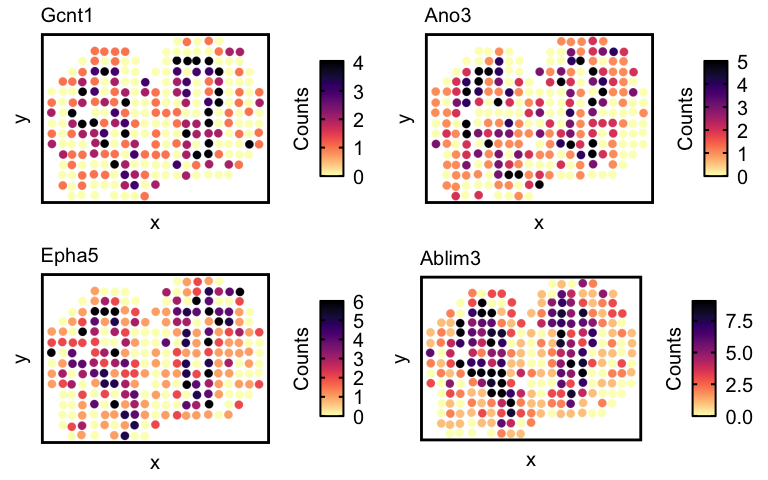Reference-free cell-type deconvolution of multi-cellular spatially resolved transcriptomics data

In this tutorial, we will walk through some of the main functionalities of `STdeconvolve`.

``````library(STdeconvolve)
``````

Given a counts matrix from pixel-resolution spatial transcriptomics data where each spatially resolved measurement may represent mixtures from potentially multiple cell-types, STdeconvolve infers the putative transcriptomic profiles of cell-types and their proportional representation within each multi-cellular spatially resolved pixel. Such a pixel-resolution spatial transcriptomics dataset of the mouse olfactory bulb is built in and can be loaded.

``````data(mOB)
pos <- mOB\$pos ## x and y positions of each pixel
cd <- mOB\$counts ## matrix of gene counts in each pixel
annot <- mOB\$annot ## annotated tissue layers assigned to each pixel
``````

`STdeconvolve` first feature selects for genes most likely to be relevant for distinguishing between cell-types by looking for highly overdispersed genes across ST pixels. Pixels with too few genes or genes with too few reads can also be removed.

``````## remove pixels with too few genes
counts <- cleanCounts(counts = cd,
min.lib.size = 100,
min.detected = 1,
verbose = TRUE)
``````
``````## Converting to sparse matrix ...

## Filtering matrix with 262 cells and 15928 genes ...

## Resulting matrix has 260 cells and 14828 genes
``````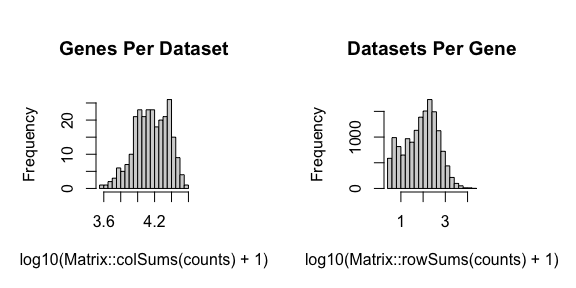``````## feature select for genes
corpus <- restrictCorpus(counts,
removeAbove = 1.0,
removeBelow = 0.05,
alpha = 0.05,
plot = TRUE,
verbose = TRUE)
``````
``````## Removing 124 genes present in 100% or more of pixels...

## 14704 genes remaining...

## Removing 3009 genes present in 5% or less of pixels...

## 11695 genes remaining...

## Restricting to overdispersed genes with alpha = 0.05...

## Calculating variance fit ...

## Using gam with k=5...

## 232 overdispersed genes ...

##  Using top 1000 overdispersed genes.

##  number of top overdispersed genes available: 232
``````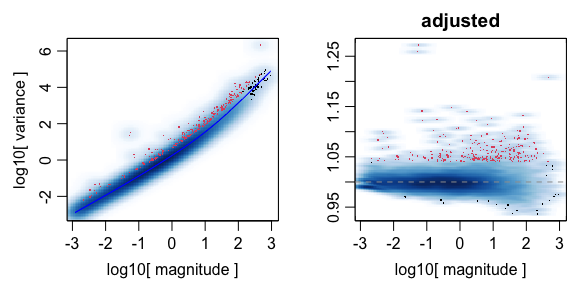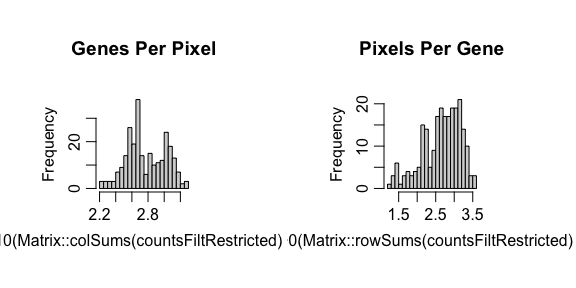`STdeconvolve` then applies latent Dirichlet allocation (LDA), a generative statistical model commonly used in natural language processing, to discover `K` latent cell-types. `STdeconvolve` fits a range of LDA models to inform the choice of an optimal `K`.

``````## Note: the input corpus needs to be an integer count matrix of pixels x genes
ldas <- fitLDA(t(as.matrix(corpus)), Ks = seq(2, 9, by = 1),
perc.rare.thresh = 0.05,
plot=TRUE,
verbose=TRUE)
``````
``````## Warning in serialize(data, node\$con): 'package:stats' may not be available

## Warning in serialize(data, node\$con): 'package:stats' may not be available

## Warning in serialize(data, node\$con): 'package:stats' may not be available

## Warning in serialize(data, node\$con): 'package:stats' may not be available

## Warning in serialize(data, node\$con): 'package:stats' may not be available

## Warning in serialize(data, node\$con): 'package:stats' may not be available

## Warning in serialize(data, node\$con): 'package:stats' may not be available

## Time to fit LDA models was 0.42 mins

## Computing perplexity for each fitted model...

## Warning in serialize(data, node\$con): 'package:stats' may not be available

## Warning in serialize(data, node\$con): 'package:stats' may not be available

## Warning in serialize(data, node\$con): 'package:stats' may not be available

## Warning in serialize(data, node\$con): 'package:stats' may not be available

## Warning in serialize(data, node\$con): 'package:stats' may not be available

## Warning in serialize(data, node\$con): 'package:stats' may not be available

## Warning in serialize(data, node\$con): 'package:stats' may not be available

## Time to compute perplexities was 0.16 mins

## Getting predicted cell-types at low proportions...

## Time to compute cell-types at low proportions was 0 mins

## Plotting...
``````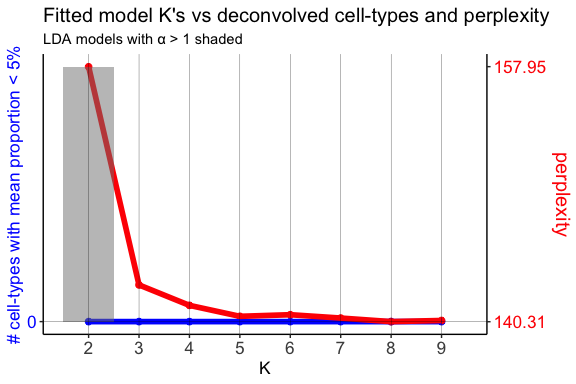In this example, we will use the model with the lowest model perplexity.

The shaded region indicates where a fitted model for a given K had an `alpha` > 1. `alpha` is an LDA parameter that is solved for during model fitting and corresponds to the shape parameter of a symmetric Dirichlet distribution. In the model, this Dirichlet distribution describes the cell-type proportions in the pixels. A symmetric Dirichlet with alpha > 1 would lead to more uniform cell-type distributions in the pixels and difficulty identifying distinct cell-types. Instead, we want models with alphas < 1, resulting in sparse distributions where only a few cell-types are represented in a given pixel.

The resulting `theta` matrix can be interpreted as the proportion of each deconvolved cell-type across each spatially resolved pixel. The resulting `beta` matrix can be interpreted as the putative gene expression profile for each deconvolved cell-type normalized to a library size of 1. This `beta` matrix can be scaled by a depth factor (ex. 1000) for interpretability.

``````## select model with minimum perplexity
optLDA <- optimalModel(models = ldas, opt = "min")

## extract pixel cell-type proportions (theta) and cell-type gene expression profiles (beta) for the given dataset
## we can also remove cell-types from pixels that contribute less than 5% of the pixel proportion
## and scale the deconvolved transcriptional profiles by 1000
results <- getBetaTheta(optLDA,
perc.filt = 0.05,
betaScale = 1000)
``````
``````## Filtering out cell-types in pixels that contribute less than 0.05 of the pixel proportion.
``````
``````deconProp <- results\$theta
deconGexp <- results\$beta
``````

We can now visualize the proportion of each deconvolved cell-type across the original spatially resolved pixels.

``````vizAllTopics(deconProp, pos,
groups = annot,
group_cols = rainbow(length(levels(annot))),
r=0.4)
``````
``````## Plotting scatterpies for 260 pixels with 8 cell-types...this could take a while if the dataset is large.
``````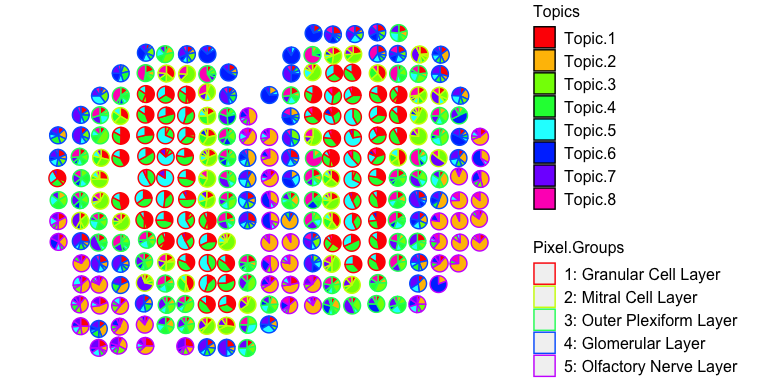For faster plotting, we can visualize the pixel proportions of a single cell-type separately using `vizTopic()`:

``````vizTopic(theta = deconProp, pos = pos, topic = "5", plotTitle = "X5",
size = 5, stroke = 1, alpha = 0.5,
low = "white",
high = "red")
``````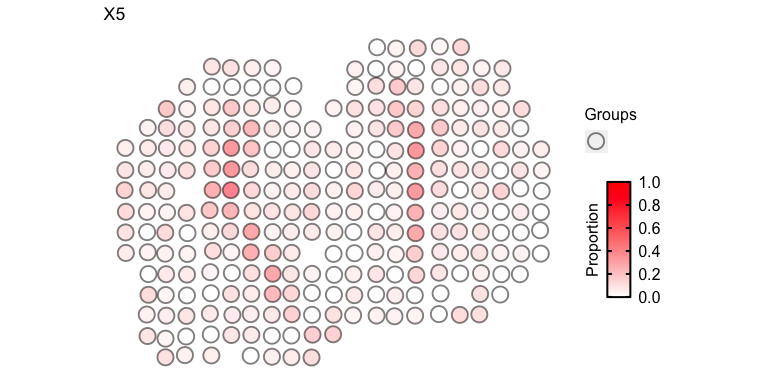We can loop through all cell-types to visualize them all together:

``````ps <- lapply(colnames(deconProp), function(celltype) {

vizTopic(theta = deconProp, pos = pos, topic = celltype, plotTitle = paste0("X", celltype),
size = 2, stroke = 1, alpha = 0.5,
low = "white",
high = "red")

})
gridExtra::grid.arrange(
grobs = ps,
layout_matrix = rbind(c(1, 2, 3),
c(4, 5, 6),
c(7, 8, 9))
)
``````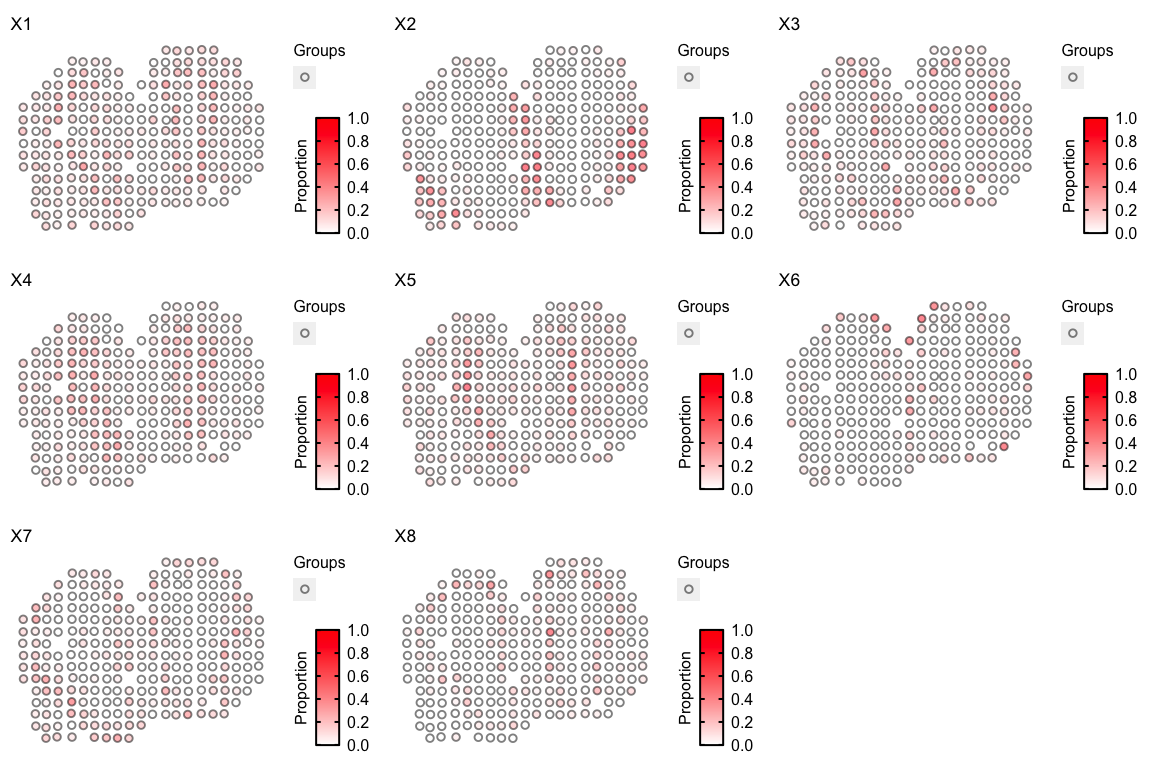We can also visualize the top marker genes for each deconvolved cell-type. We will use deconvolved cell-types `5` and `1` as examples here. We will define the top marker genes here as genes highly expressed in the deconvolved cell-type (count > 5) that also have the top 4 highest log2(fold change) when comparing the deconvolved cell-type’s expression profile to the average of all other deconvolved cell-types’ expression profiles.

``````celltype <- 5
## highly expressed in cell-type of interest
highgexp <- names(which(deconGexp[celltype,] > 5))
## high log2(fold-change) compared to other deconvolved cell-types
log2fc <- sort(log2(deconGexp[celltype,highgexp]/colMeans(deconGexp[-celltype,highgexp])), decreasing=TRUE)
markers <- names(log2fc)[1:4]

# -----------------------------------------------------
## visualize the transcriptional profile
dat <- data.frame(values = as.vector(log2fc), genes = names(log2fc), order = seq(length(log2fc)))
# Hide all of the text labels.
dat\$selectedLabels <- ""
dat\$selectedLabels[1:4] <- markers

plt <- ggplot2::ggplot(data = dat) +
ggplot2::geom_col(ggplot2::aes(x = order, y = values,
fill = factor(selectedLabels == ""),
color = factor(selectedLabels == "")), width = 1) +

ggplot2::scale_fill_manual(values = c(STdeconvolve::transparentCol("darkblue", percent = 0),
STdeconvolve::transparentCol("darkblue", percent = 0)
)) +
ggplot2::scale_color_manual(values = c(STdeconvolve::transparentCol("darkblue", percent = 0),
STdeconvolve::transparentCol("darkblue", percent = 0)
)) +

ggplot2::scale_y_continuous(expand = c(0, 0), limits = c(min(log2fc) - 0.3, max(log2fc) + 0.3)) +
ggplot2::scale_x_continuous(expand = c(0, 0), limits = c(-2, NA)) +

ggplot2::labs(title = "Deconvolved cell-type X5 transcriptional profile",
x = "Gene expression rank",
y = "log2(FC)") +

ggplot2::geom_text(ggplot2::aes(x = order, y = values, label = selectedLabels), color = "red") +

ggplot2::theme_classic() +
ggplot2::theme(axis.text.x = ggplot2::element_text(size=15, color = "black"),
axis.text.y = ggplot2::element_text(size=15, color = "black"),
axis.title.y = ggplot2::element_text(size=15, color = "black"),
axis.title.x = ggplot2::element_text(size=15, color = "black"),
axis.ticks.x = ggplot2::element_blank(),
plot.title = ggplot2::element_text(size=15),
legend.text = ggplot2::element_text(size = 15, colour = "black"),
legend.title = ggplot2::element_text(size = 15, colour = "black", angle = 90),
panel.background = ggplot2::element_blank(),
plot.background = ggplot2::element_blank(),
panel.grid.major.y = ggplot2::element_line(size = 0.3, colour = STdeconvolve::transparentCol("gray80", percent = 0)),
axis.line = ggplot2::element_line(size = 1, colour = "black"),
legend.position="none"
)
plt
``````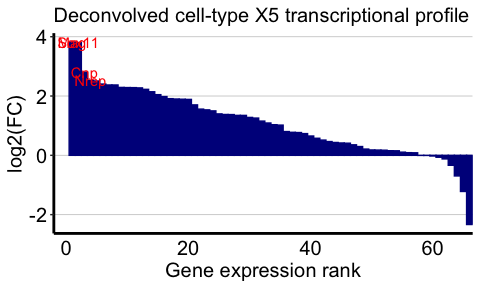Now lets visualize the spatial expression of the top 4 genes.

``````## visualize spatial expression of top genes
df <- merge(as.data.frame(pos),
as.data.frame(t(as.matrix(counts[markers,]))),
by = 0)
ps <- lapply(markers, function(marker) {
vizGeneCounts(df = df,
gene = marker,
# groups = annot,
# group_cols = rainbow(length(levels(annot))),
size = 3, stroke = 0.1,
plotTitle = marker,
winsorize = 0.05,
showLegend = TRUE) +

## remove the pixel "groups", which is the color aesthetic for the pixel borders
ggplot2::guides(colour = "none") +

## change some plot aesthetics
ggplot2::theme(axis.text.x = ggplot2::element_text(size=0, color = "black", hjust = 0, vjust = 0.5),
axis.text.y = ggplot2::element_text(size=0, color = "black"),
axis.title.y = ggplot2::element_text(size=15),
axis.title.x = ggplot2::element_text(size=15),
plot.title = ggplot2::element_text(size=15),
legend.text = ggplot2::element_text(size = 15, colour = "black"),
legend.title = ggplot2::element_text(size = 15, colour = "black", angle = 90),
panel.background = ggplot2::element_blank(),
## border around plot
panel.border = ggplot2::element_rect(fill = NA, color = "black", size = 2),
plot.background = ggplot2::element_blank()
) +
ggplot2::guides(fill = ggplot2::guide_colorbar(title = "Counts",
title.position = "left",
title.hjust = 0.5,
ticks.colour = "black",
ticks.linewidth = 2,
frame.colour= "black",
frame.linewidth = 2,
label.hjust = 0
))
})
gridExtra::grid.arrange(
grobs = ps,
layout_matrix = rbind(c(1, 2),
c(3, 4))
)
``````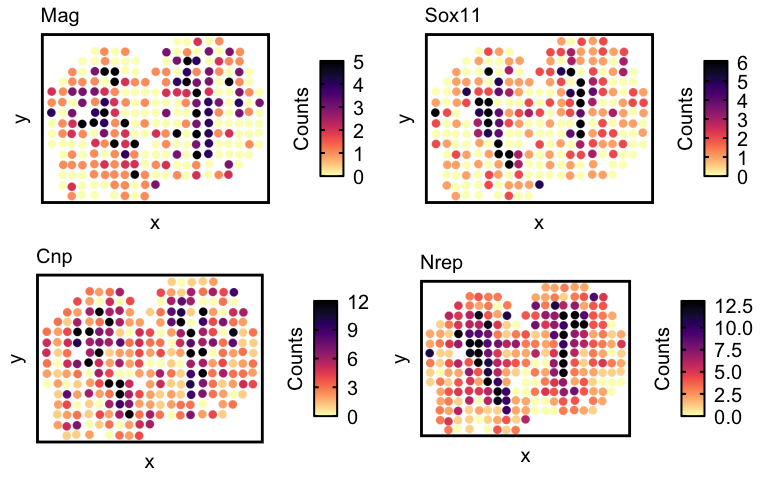And now for cell-type 1:

``````celltype <- 1
## highly expressed in cell-type of interest
highgexp <- names(which(deconGexp[celltype,] > 5))
## high log2(fold-change) compared to other deconvolved cell-types
log2fc <- sort(log2(deconGexp[celltype,highgexp]/colMeans(deconGexp[-celltype,highgexp])), decreasing=TRUE)
markers <- names(log2fc)[1:4]

# -----------------------------------------------------
## visualize the transcriptional profile
dat <- data.frame(values = as.vector(log2fc), genes = names(log2fc), order = seq(length(log2fc)))
# Hide all of the text labels.
dat\$selectedLabels <- ""
dat\$selectedLabels[1:4] <- markers

plt <- ggplot2::ggplot(data = dat) +
ggplot2::geom_col(ggplot2::aes(x = order, y = values,
fill = factor(selectedLabels == ""),
color = factor(selectedLabels == "")), width = 1) +

ggplot2::scale_fill_manual(values = c(STdeconvolve::transparentCol("darkblue", percent = 0),
STdeconvolve::transparentCol("darkblue", percent = 0)
)) +
ggplot2::scale_color_manual(values = c(STdeconvolve::transparentCol("darkblue", percent = 0),
STdeconvolve::transparentCol("darkblue", percent = 0)
)) +

ggplot2::scale_y_continuous(expand = c(0, 0), limits = c(min(log2fc) - 0.3, max(log2fc) + 0.3)) +
ggplot2::scale_x_continuous(expand = c(0, 0), limits = c(-2, NA)) +

ggplot2::labs(title = "Deconvolved cell-type X1 transcriptional profile",
x = "Gene expression rank",
y = "log2(FC)") +

ggplot2::geom_text(ggplot2::aes(x = order, y = values, label = selectedLabels), color = "red") +

ggplot2::theme_classic() +
ggplot2::theme(axis.text.x = ggplot2::element_text(size=15, color = "black"),
axis.text.y = ggplot2::element_text(size=15, color = "black"),
axis.title.y = ggplot2::element_text(size=15, color = "black"),
axis.title.x = ggplot2::element_text(size=15, color = "black"),
axis.ticks.x = ggplot2::element_blank(),
plot.title = ggplot2::element_text(size=15),
legend.text = ggplot2::element_text(size = 15, colour = "black"),
legend.title = ggplot2::element_text(size = 15, colour = "black", angle = 90),
panel.background = ggplot2::element_blank(),
plot.background = ggplot2::element_blank(),
panel.grid.major.y = ggplot2::element_line(size = 0.3, colour = STdeconvolve::transparentCol("gray80", percent = 0)),
axis.line = ggplot2::element_line(size = 1, colour = "black"),
legend.position="none"
)
plt
``````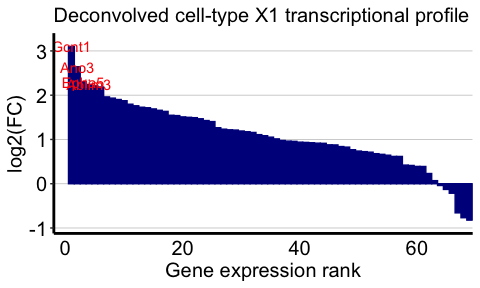And the spatial expression of the top genes:

``````## visualize spatial expression of top genes
df <- merge(as.data.frame(pos),
as.data.frame(t(as.matrix(counts[markers,]))),
by = 0)
ps <- lapply(markers, function(marker) {
vizGeneCounts(df = df,
gene = marker,
# groups = annot,
# group_cols = rainbow(length(levels(annot))),
size = 3, stroke = 0.1,
plotTitle = marker,
winsorize = 0.05,
showLegend = TRUE) +

## remove the pixel "groups", which is the color aesthetic for the pixel borders
ggplot2::guides(colour = "none") +

## change some plot aesthetics
ggplot2::theme(axis.text.x = ggplot2::element_text(size=0, color = "black", hjust = 0, vjust = 0.5),
axis.text.y = ggplot2::element_text(size=0, color = "black"),
axis.title.y = ggplot2::element_text(size=15),
axis.title.x = ggplot2::element_text(size=15),
plot.title = ggplot2::element_text(size=15),
legend.text = ggplot2::element_text(size = 15, colour = "black"),
legend.title = ggplot2::element_text(size = 15, colour = "black", angle = 90),
panel.background = ggplot2::element_blank(),
## border around plot
panel.border = ggplot2::element_rect(fill = NA, color = "black", size = 2),
plot.background = ggplot2::element_blank()
) +
ggplot2::guides(fill = ggplot2::guide_colorbar(title = "Counts",
title.position = "left",
title.hjust = 0.5,
ticks.colour = "black",
ticks.linewidth = 2,
frame.colour= "black",
frame.linewidth = 2,
label.hjust = 0
))
})
gridExtra::grid.arrange(
grobs = ps,
layout_matrix = rbind(c(1, 2),
c(3, 4))
)
``````